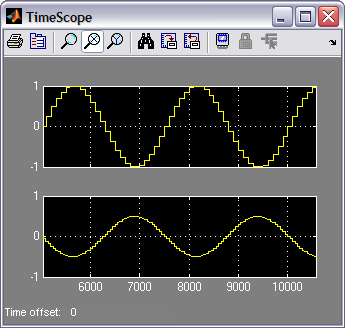ID 683337
Date 5/01/2023
Public

Give Feedback

## 13.1.9. Interpolating FIR

The InterpolatingFIR block implements a highly efficient multichannel FIR filter across a broad range of parameters directly from a Simulink model. A memory-mapped interface allows you to read and write coefficients directly, easing system integration. The InterpolatingFIR block performs filtering on a stream of multichannel input data and produces a stream of output data with increased sampling frequency.

You can use the InterpolatingFIR block in a digital up converter for a radio system or a general purpose DSP application. The coefficients and input data are fixed-point types, and the output is the implied full precision fixed-point type. You can reduce the precision by using a separate Scale block, which can perform rounding and saturation to provide the required output precision.

The InterpolatingFIR block supports:

• Rate changes from two upwards
• Coefficient width in bits from 2 to 32 bits
• Data output width in bits from 4 to 64 bits
• Half-band and L-band Nyquist filters
• Symmetry and anti(negative)-symmetry
• Real filters

In the basic equation, at each sample time k, the new output y, is calculated by multiplying coefficients a, by the recent past values of the input x.

The InterpolatingFIR has a higher output sample rate than the input sample rate by a factor, I, the interpolation factor. Usually, the interpolating FIR inserts I–1 zeroes for every input sample, thus raising the sample rate by a factor I.

The physical implementation avoids performing multiplications with these zero samples, reducing the filter cost.

Figure 105. Interpolate by 2 Filter Increasing Sample Rate of a Sine Wave InputTable 48.  Parameters for the InterpolatingFIR Block
Parameter Description
Input rate per channel Specifies the sampling frequency of the input data per channel measured in millions of samples per second (MSPS).
Interpolation Specifies the interpolation rate. Must be an integer.
Number of channels Specifies the number of unique channels to process.
Symmetry You can select Symmetrical or Anti-Symmetrical coefficients. Symmetrical coefficients can result in hardware resource savings over the asymmetrical version.
Coefficients You can specify the filter coefficients using a Simulink fixed-point object fi(0). The data type of the fixed-point object determines the width and format of the coefficients. The length of the array determines the length of the filter.

For example, fi(fir1(49, 0.3),1,18,19).

Base address You can memory map the filter's coefficients into the address space of the system. This field determines the starting address for the coefficients. It is specified as a MATLAB double type (decimal integer) but you can use a MATLAB expression to specify a hexadecimal or octal type if required.
Read/Write mode You can allow Read, Write, or Read/Write access from the system interface. Turn on Constant. to map coefficients to the system address space.
Filter structure You can select Use All Taps, Half Band, or a specified band (from 3rd Band to 46th Band).
Expose Avalonmemory-mapped agent in Simulink Allows you to reconfigure coefficients without Platform Designer. Also, it allows you to reprogram multiple FIR filters simultaneously. Turn on to show the Avalon-MM inputs and outputs as normal ports in Simulink. The Read/Write mode decides the valid subset of Avalon memory-mapped agent ports that appear on the block. If you select Constant, the block shows no Avalon-MM ports.
Reconfigurable channels Turn on for a reconfigurable FIR filter.
Channel mapping Enter parameters as a MATLAB 2D aray for reconfigurable FIR filter. Each row represents a mode; each entry in a row represents the channel input on that time slot. For example, [0,0,0,0;0,1 2,3] gives the first element of the second row as 0, which means DSP Builder processes channel 0 on the first cycle when the FIR is set to mode 1.
Table 49.  Port Interface for the InterpolatingFIR Block
Signal Direction Description
a Input The fixed-point data input to the block. If you request more channels than can fit on a single bus, this signal is a vector. The width in bits is inherited from the input wire.
v Input Indicates validity of the data input signals. If v is high, the data on the a wire is valid.
c Input Indicates the channel of the data input signals. If v is high, c indicates which channel the data corresponds to.
m Input Indicates reconfigurable filter.
b Input Indicates multibank filter. This input appears when you add a second filter definition to the Coefficients parameter in the parameters dialog box.
q Output The fixed-point filtered data output from the block. If you request more channels than can fit on a single bus, this signal is a vector. The width in bits is a function of the input width in bits and the parameterization.
v Output Indicates validity of data output signals. The output data can be non-zero when v is low
c Output Indicates the channel of the data output signals. The output data can be non-zero when v is low